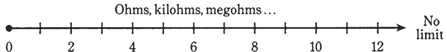## Inductive reactance Assignment Help

Assignment Help: >> Electrical Engineering - Inductive reactance

Inductive reactance:

IN DIRECT CURRENT CIRCUITS, RESISTANCE  IS A SIMPLE THING. THIS CAN BE EXPRESSED AS A number, from zero  to large values, increasing without limit through the millions, and even trillions of ohms. Physicists resistance is a scalar quantity, as it can be expressed on a 1-dimensional scale. Actually, direct current resistance can be represented along a half line, or ray, as shown in the Figure given below.Figure--   Resistance can be represented on a ray.

Given a certain direct current voltage, the current decreases as resistance increases, in accordance  with Ohm's Law, as you know already. The same law holds for alternating current through a resistance, if the alternating current voltage and current are both specified as peak, peak to peak, or RMS values.

 Coils and alternating current Coils and direct current Current lags voltage Pictorial method Points in the RL plane Pure resistance Reactance and frequency Trigonometric method of lag Vectors in the RL Plane